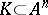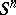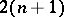# Helly's theorem

(diff) ← Older revision | Latest revision (diff) | Newer revision → (diff)

Helly's theorem on the intersection of convex sets with a common point: Letbe a family of at leastconvex sets in an-dimensional affine space, where eitheris finite or each set inis compact; if eachsets of the family have a common point, then there is a point that is common to all the sets of.

Many studies are devoted to Helly's theorem, concerning applications of it, proofs of various analogues, and propositions similar to Helly's theorem generalizing it, for example, in problems of Chebyshev approximation, in the solution of the illumination problem, and in the theory of convex bodies (cf. Convex body). Frequently, Helly's theorem figures in proofs of combinatorial propositions of the following type: If in a certain family each subfamily ofterms has a certain property, then the whole family has this property. For example, ifandare two points of a set, then the expression "a is visible from b in K" means that the segmentbelongs to. Suppose that a compact sethas the property that for anypoints inthere is a point infrom which all these points are visible; then there is a point infrom which all the points ofare visible, that is,is a star-shaped set.

The majority of analogues of Helly's theorem and its generalizations are connected with various versions of the concept of "convexity" . For example, letbe a Euclidean sphere; a set is called convex in the sense of Robinson if, together with every pair of points that are not diametrically opposite, it contains the smaller arc joining these points of the great circle defined by them. If a family of closed sets ofthat are convex in the sense of Robinson is such that anyelements of it have a non-empty intersection, then all the elements of this family have a non-empty intersection.

Helly's theorem was established by E. Helly in 1913.

## Contents

How to Cite This Entry:
Helly's theorem. Encyclopedia of Mathematics. URL: http://encyclopediaofmath.org/index.php?title=Helly%27s_theorem&oldid=16299
This article was adapted from an original article by P.S. Soltan (originator), which appeared in Encyclopedia of Mathematics - ISBN 1402006098. See original article